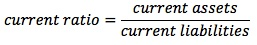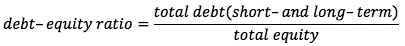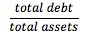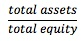### Why should I choose AnalystNotes?

Simply put: AnalystNotes offers the best value and the best product available to help you pass your exams.

##### Subject 5. Uses and Analysis of the Balance Sheet

Common-Size Analysis of Balance Sheets

This topic will be discussed in detail in Reading 20 [Financial Analysis Techniques].

Balance Sheet Ratios

Liquidity ratios measure the ability of a company to meet future short-term financial obligations from current assets and, more importantly, cash flows. Each of the following ratios takes a slightly different view of cash or near-cash items.

• Current Ratio is a measure of the number of dollars of current assets available to meet current obligations. It is the best-known liquidity measure. A current ratio of less than 1 indicates the company has negative working capital.• Quick Ratio (Acid-Test Ratio) eliminates less liquid assets, such as inventory and pre-paid expenses, from the current ratio. If inventory is not moving, the quick ratio is a better indicator of cash and near-cash items that will be available to meet current obligations.• Cash Ratio is the most conservative liquidity ratio, determined by eliminating receivables from the quick ratio. As with the elimination of inventory in the quick ratio, there is no guarantee that the receivables will be collected.Solvency ratios measure a company's ability to meet long-term and other obligations.

• Long-Term Debt-Equity Ratio is an indicator of the degree of protection available to the creditors in the event of insolvency of a company. Higher debt-equity ratio indicates higher financial risk.• Debt-Equity Ratio includes short-term debt in the numerator.The total debt includes all liabilities, including non-interest-bearing debt such as accounts payables, accrued expenses, and deferred taxes. This ratio is especially useful in analyzing a company with substantial financing from short-term borrowing.

• Total Debt Ratio =• Financial Leverage Ratio =Financial statement analysis aims to investigate a company's financial condition and operating performance. Using financial ratios helps to examine relationships among individual data items from financial statements. Although ratios by themselves cannot answer questions, they can help analysts ask the right questions in financial statement analysis. As analytical tools, ratios are attractive because they are simple and convenient. However, ratios are only as good as the data upon which they are based and the information with which they are compared.

From the earlier discussion it is obvious that there are a significant number of estimates and subjective information that go into financial statements and therefore it is imperative that the end user understands the numbers before calculating and relying on ratio analyses based on these numbers.

User Comment
omya Quick Ratio/ Acid Test Ratio - doesnt include Inventories.
Cash Ratio - doesnt include debtors and inventories.
Solvency ratios - for meeting long term debt obligations.
Debt Equity Ratio- here debt means short term + long term debts both. Here non interest bearing debts are also considered. i.e. accounts payable..
FInancial Leverage Ratio = Total Assets/Total Equity.
farhan92 issue with fin lev is that you would assume theres debt involved in the formula